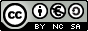Multiples and factors. Prime numbers First and Second Year of Secondary Education

The multiples of a number.

The multiples of a natural number are the natural numbers you get when you multiply that number by other natural numbers. We say that a number is a multiple of another number when it contains this other number a whole number of times.

Esta unidad interactiva requiere la máquina virtual de Java J2RE.
 Find the numbers below that are multiples of the numbers above and take them to the rectangle on the left. Take the numbers that are not multiples to the rectangle on the right.

In order to do this, you can do the division mentally or use the divisibility criteria.

- The number 0 has only one multiple, that is 0. The rest of the natural numbers has an infinite number of multiples. The number 0 is a multiple of all the numbers.

- All the numbers are multiples of 1.

- The multiples of 2 end in 0, 2, 4, 6, 8.

- In the multiples of 3, the sum of its digits is also a multiple of 3.

- The multiples of 5 end in 0 or in 5.

- The multiples of 6 end in 0, 2, 4, 6, 8 and the sum of its digits is a multiple of 3.

- In the multiples of 9, the sum of its digits is a multiple of 9.

 Eduardo Barbero CorralSpanish Ministry of Education. Year 2007